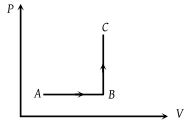# NEET Physics Thermodynamics Questions Solved

The P-V diagram of a system undergoing thermodynamic transformation is shown in figure. The work done by the system in going from AB C is 30J and 40J heat is given to the system. The change in internal energy between A and C is(1) 10 J

(2) 70 J

(3) 84 J

(4) 134 J

(1) Heat given ΔQ = 40 J and Work done ΔW = 30 J

⇒ ΔU = ΔQ – ΔW = 40 – 30 = 10 J.

Difficulty Level:

• 39%
• 41%
• 17%
• 4%